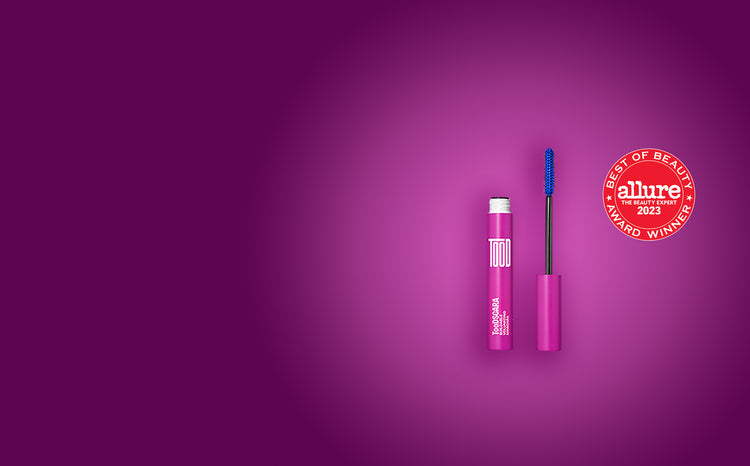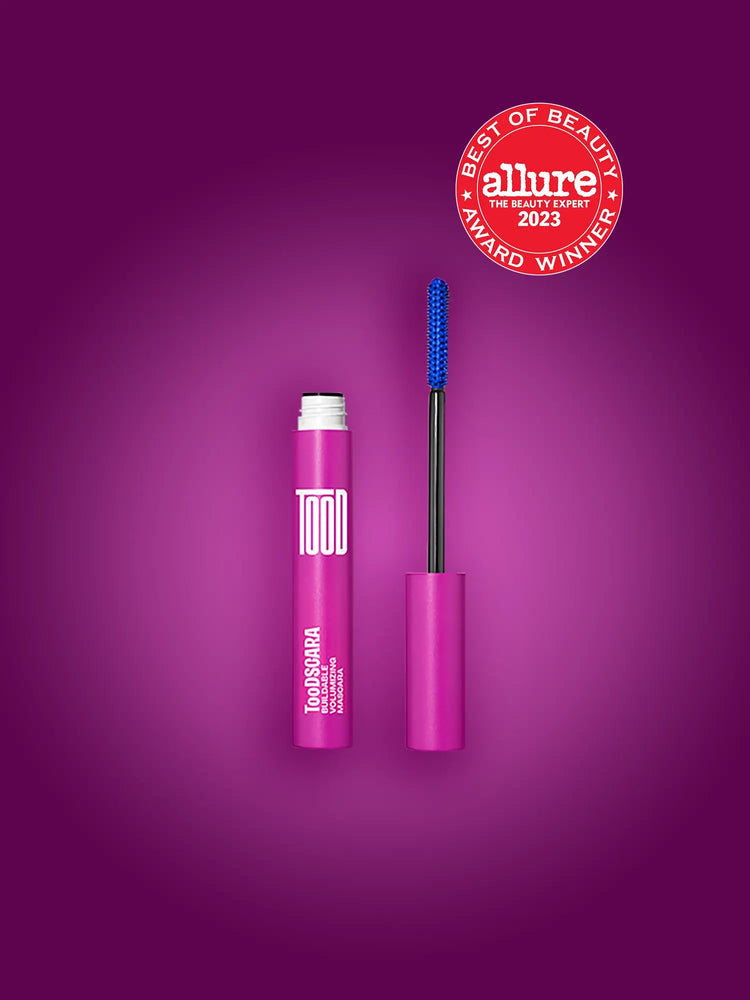# Allure Best of Beauty 2023 Award Winner for Mascara.

• we're cracking the code on clean formulas • we're cracking the code on clean formulas • we're cracking the code on clean formulas • we're cracking the code on clean formulas • we're cracking the code on clean formulas • we're cracking the code on clean formulas • we're cracking the code on clean formulas • we're cracking the code on clean formulas
• we're cracking the code on clean formulas • we're cracking the code on clean formulas • we're cracking the code on clean formulas • we're cracking the code on clean formulas • we're cracking the code on clean formulas • we're cracking the code on clean formulas • we're cracking the code on clean formulas • we're cracking the code on clean formulas